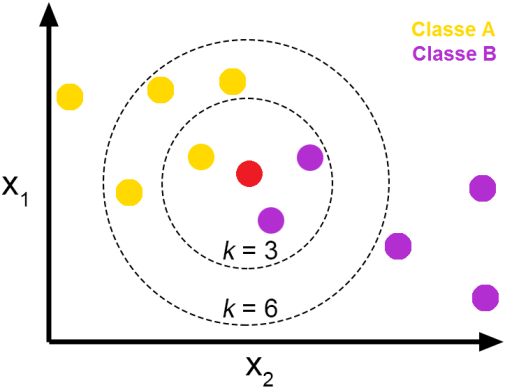# K-Nearest Neighbors (KNN) Algorithm

A Brief IntroductionSimple Analogy for K-Nearest Neighbors (K-NN)

In this blog, we’ll talk about one of the most widely used machine learning algorithms for classification, which is the K-Nearest Neighbors (KNN) algorithm. K-Nearest Neighbor (K-NN) is a simple, easy to understand, versatile and one of the topmost machine learning algorithms that find its applications in a variety of fields.

We’ll try to understand what is KNN, how it works, some common distance metrics used in KNN, its advantages & disadvantages along with some of its modern applications.

## What is K-NN ?

K-NN is a non-parametric and lazy learning algorithm. Non-parametric means there is no assumption for underlying data distribution i.e. the model structure is determined from the dataset.

It is called Lazy algorithm because it does not need any training data points for model generation. All training data is used in the testing phase which makes training faster and testing phase slower and costlier.

K-Nearest Neighbor (K-NN) is a simple algorithm that stores all the available cases and classifies the new data or case based on a similarity measure.

## K-NN classification

In K-NN classification, the output is a class membership. An object is classified by a plurality vote of its neighbors, with the object being assigned to the class most common among its k nearest neighbors (k is a positive integer, typically small). If k = 1, then the object is simply assigned to the class of that single nearest neighbor.

To determine which of the K instances in the training dataset are most similar to a new input, a distance measure is used. For real-valued input variables, the most popular distance measure is the Euclidean distance.The Red point is classified to the class most common among its k nearest neighbors

## The Euclidean distance

• The Euclidean distance is the most common distance metric used in low dimensional data sets. It is also known as the L2 norm. The Euclidean distance is the usual manner in which distance is measured in the real world.

where p and q are n-dimensional vectors and denoted by p = (p1, p2,…, pn) and q = (q1, q2,…, qn) represent the n attribute values of two records.

• While Euclidean distance is useful in low dimensions, it doesn’t work well in high dimensions and for categorical variables. The drawback of Euclidean distance is that it ignores the similarity between attributes. Each attribute is treated as totally different from all of the attributes.
• Hamming Distance: Calculate the distance between binary vectors.
• Manhattan Distance: Calculate the distance between real vectors using the sum of their absolute difference. Also called City Block Distance.
• Minkowski Distance: Generalization of Euclidean and Manhattan distance.

## Steps to be carried out during the K-NN algorithm are as follows :

1. Divide the data into training and test data.
2. Select a value K.
3. Determine which distance function is to be used.
4. Choose a sample from the test data that needs to be classified and compute the distance to its n training samples.
5. Sort the distances obtained and take the k-nearest data samples.
6. Assign the test class to the class based on the majority vote of its k neighbors.

## Performance of the K-NN algorithm is influenced by three main factors :

1. The distance function or distance metric used to determine the nearest neighbors.
2. The decision rule used to derive a classification from the K-nearest neighbors.
3. The number of neighbors used to classify the new example.

## Advantages of K-NN :

1. The K-NN algorithm is very easy to implement.
2. Nearly optimal in the large sample limit.
3. Uses local information, which can yield highly adaptive behavior.
4. Lends itself very easily to parallel implementation.

## Disadvantages of K-NN :

1. Large storage requirements.
2. Computationally intensive recall.
3. Highly susceptible to the curse of dimensionality.

## K-NN Algorithm finds its applications in :

1. Finance — financial institutes will predict the credit rating of customers.
2. Healthcare — gene expression.
3. Political Science — classifying potential voters in two classes will vote or won’t vote.
4. Handwriting detection.
5. Image Recognition.
6. Video Recognition.
7. Pattern Recognition.